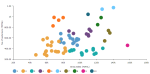# DCPY.MEANSHIFTCLUST(cluster_all, bandwidth, columns)

Mean shift is a clustering algorithm that assigns the data points to the clusters iteratively by shifting points towards the mode. The mode can be understood as the highest density of data points in the region. As such, it is also known as the mode-seeking algorithm. Given a set of data points, the algorithm iteratively assigns each data point towards the closest cluster centroid. Each iteration will move each data point closer to where the most points are at, which is or will lead to the cluster center.

###### Parameters
• cluster_all – If True, then all points are clustered: even the orphans that are not within any kernel are assigned to the nearest one. If False, orphans will have label -1, Boolean (default True).

• bandwidth – Bandwidth used in kernel density estimation. Lower number results in higher number of clusters and vice versa. When None, it is estimated automatically, float (default None).

• columns – Dataset columns or custom calculations.

Example:DCPY.MEANSHIFTCLUST(True, 0.7, sum([Gross Sales]), sum([No of customers])) used as a calculation for the Color field of the Scatterplot visualization.###### Input data
• Numeric variables are automatically scaled to zero mean and unit variance.
• Character variables are transformed to numeric values using one-hot encoding.
• Dates are treated as character variables, so they are also one-hot encoded.
• Size of input data is not limited, but many categories in character or date variables increase rapidly the dimensionality.
• Rows that contain missing values in any of their columns are dropped.
###### Result
• Column of integer values starting with 0, where each number corresponds to a cluster assigned to each record (row) by the algorithm, -1 for orphans if False is specified in cluster_all parameter.
• Rows that were dropped from input data due to containing missing values have missing value instead of assigned cluster.
###### Key usage points
• Use it when you expect to find relatively higher number of clusters with uneven size or shape.
• Estimates the optimum number of clusters.
• Not scalable with number of records.
• Often fails to find appropriate clusters for outliers that are located between natural clusters in lower density regions.
• Higher sensitivity to initialization.
###### Example

For the whole list of algorithms, see Data science built-in algorithms.